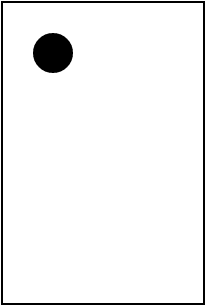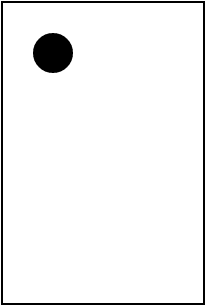# Timers

Animation is a key aspect of many types of computer programs. This is especially true of games. For example, you'll to create a game like Breakout, you'll need to make use of animations to bounce a ball around the screen and move the player's paddle. A good understanding how animation works will be beneficial as you design and build more complex programs.

## The Idea of Animation

Animation can be broken down into three basic steps:

• Draw
• Wait and Move
• Repeat

You may recall creating flipbook animations in the corner of a notebook -- this is essentially the same process. Each page of a flipbook draws a similar image that is moved slightly. Flipping rapidly through the pages of the flip book causes the image to appear to move. This is one of the earliest forms of animation, known originally as the kineograph.We can explore the basics of animation by having a circle move across the canvas from top to bottom.

#### Draw

Drawing is how elements are displayed on the canvas. As you learned in the previous chapter, an element is first created and given a position, then it is added to the canvas.

In this case, we will draw a black circle at position `(50, 50)`.

var ball;

``````function start(){
ball = new Circle(20);
ball.setPosition(50, 50);
}
``````#### Wait and Move

Now that the ball is on the screen, we want to wait a moment, then move the ball toward the bottom of the canvas. If we moved the ball right away without waiting, then the user would only see the ball after it has been moved. This delay is essential to creating the animation effect.

We can move the ball with the `move(x, y)` method. This method will move the object `x` pixels in the x direction, and `y` pixels in the y direction. In our case, we want the ball to fall straight down, so we will have `x` change by 0 and `y` change by 25.

``````ball.move(0, 25);
``````

But how do we have the program wait before moving the ball? And what about repeating the process? A loop won't quite work, as there's no way to have a loop pause between iterations. We'll need to use a new tool, the timer, to create our animation.

## Introducing Timers

Timers allow us to call a specified function repeatedly with a pause, or delay, between each function call. Timers and loops are similar in that they both repeate code, but they are actually quite different. A loop has no sense of time or delay -- it enters the next iteration as soon as one iteration finishes. A timer calls a function at specific intervals until the programmer tells the timer to stop.

The ability to call a function over and over with a pause in between is very helpful when creating animation. For example, the code above to move the ball can be placed into a `draw` function:

``````function draw(){
ball.move(0, 25);
}
``````

We can then set a timer in `start` to call this `draw` function every 75 milliseconds. The full program looks like this:

``````var ball;

function start(){
ball = new Circle(20);
ball.setPosition(50, 50);

setTimer(draw, 75);
}

function draw(){
ball.move(0, 25);
}
``````

This will move the position of the ball 25 pixels down every 75 milliseconds. We can track the position of the circle each tick of the timer:

Timer iteration Overall time (milliseconds) (x, y)
0 0 (50, 50)
1 75 (50, 75)
2 150 (50, 100)
2 225 (50, 125)
4 300 (50, 150)
5 375 (50, 175)
6 450 (50, 200)
7 525 (50, 225)
8 600 (50, 250)
9 675 (50, 275)
10 750 (50, 300)
11 825 (50, 325)

This results in the following animation of the ball falling: